Anzeige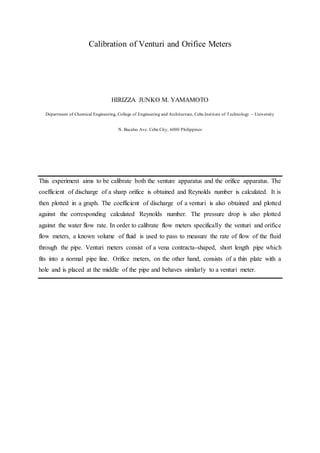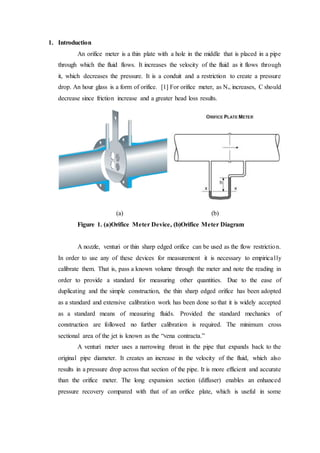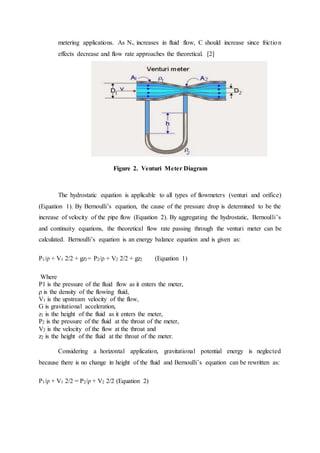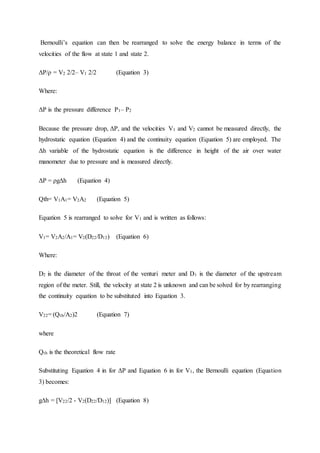Anzeige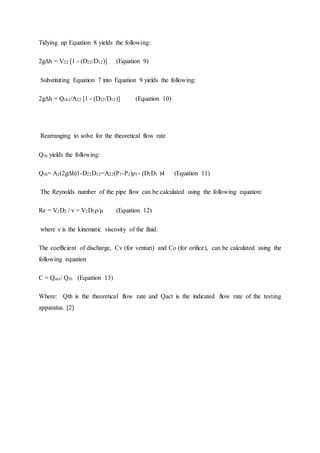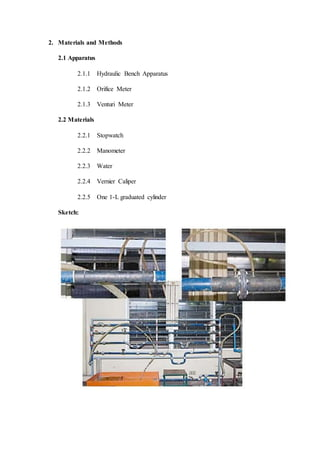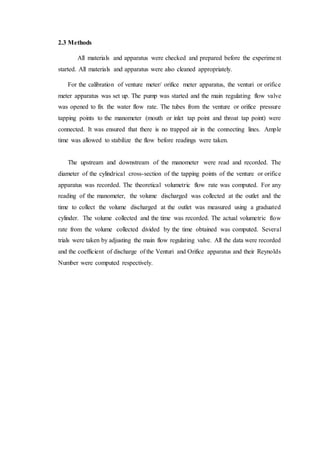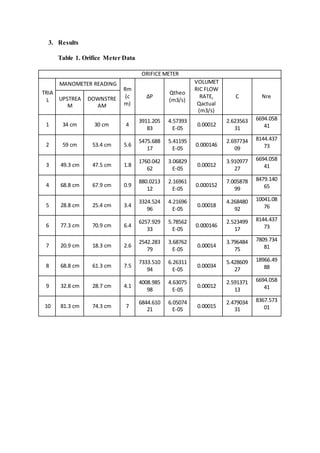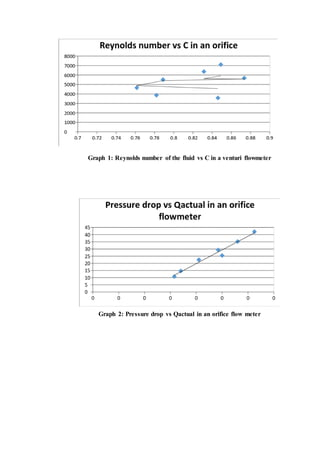Anzeige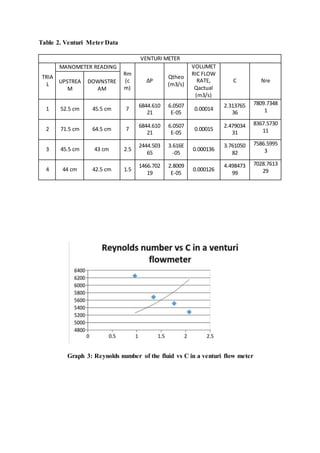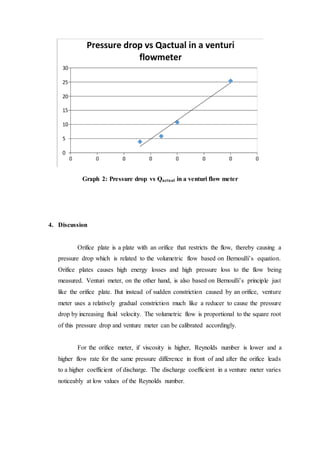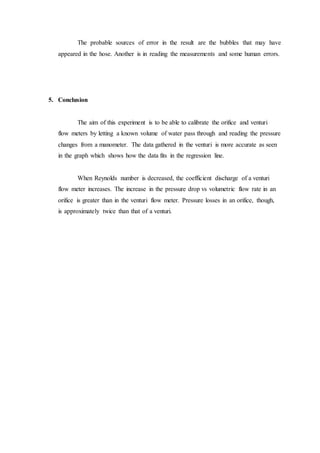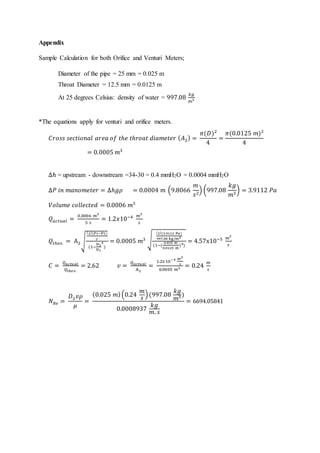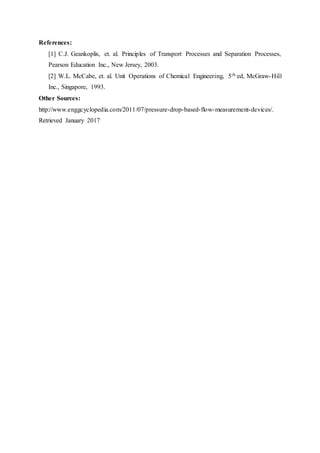Nächste SlideShare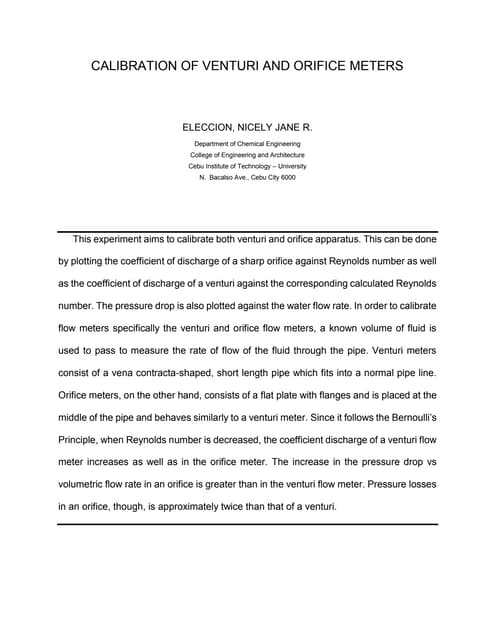Calibration of Venturi and Orifice Meters
1 von 14
Anzeige

### Calibration of venturi and orifice meters

1. Calibration of Venturi and Orifice Meters HIRIZZA JUNKO M. YAMAMOTO Department of Chemical Engineering, College of Engineering and Architecture, Cebu Institute of Technology – University N. Bacalso Ave. Cebu City, 6000 Philippines This experiment aims to be calibrate both the venture apparatus and the orifice apparatus. The coefficient of discharge of a sharp orifice is obtained and Reynolds number is calculated. It is then plotted in a graph. The coefficient of discharge of a venturi is also obtained and plotted against the corresponding calculated Reynolds number. The pressure drop is also plotted against the water flow rate. In order to calibrate flow meters specifically the venturi and orifice flow meters, a known volume of fluid is used to pass to measure the rate of flow of the fluid through the pipe. Venturi meters consist of a vena contracta-shaped, short length pipe which fits into a normal pipe line. Orifice meters, on the other hand, consists of a thin plate with a hole and is placed at the middle of the pipe and behaves similarly to a venturi meter.
2. 1. Introduction An orifice meter is a thin plate with a hole in the middle that is placed in a pipe through which the fluid flows. It increases the velocity of the fluid as it flows through it, which decreases the pressure. It is a conduit and a restriction to create a pressure drop. An hour glass is a form of orifice.  For orifice meter, as NRe increases, C should decrease since friction increase and a greater head loss results. (a) (b) Figure 1. (a)Orifice Meter Device, (b)Orifice Meter Diagram A nozzle, venturi or thin sharp edged orifice can be used as the flow restriction. In order to use any of these devices for measurement it is necessary to empirically calibrate them. That is, pass a known volume through the meter and note the reading in order to provide a standard for measuring other quantities. Due to the ease of duplicating and the simple construction, the thin sharp edged orifice has been adopted as a standard and extensive calibration work has been done so that it is widely accepted as a standard means of measuring fluids. Provided the standard mechanics of construction are followed no further calibration is required. The minimum cross sectional area of the jet is known as the “vena contracta.” A venturi meter uses a narrowing throat in the pipe that expands back to the original pipe diameter. It creates an increase in the velocity of the fluid, which also results in a pressure drop across that section of the pipe. It is more efficient and accurate than the orifice meter. The long expansion section (diffuser) enables an enhanced pressure recovery compared with that of an orifice plate, which is useful in some
3. metering applications. As NRe increases in fluid flow, C should increase since friction effects decrease and flow rate approaches the theoretical.  Figure 2. Venturi Meter Diagram The hydrostatic equation is applicable to all types of flowmeters (venturi and orifice) (Equation 1). By Bernoulli’s equation, the cause of the pressure drop is determined to be the increase of velocity of the pipe flow (Equation 2). By aggregating the hydrostatic, Bernoulli’s and continuity equations, the theoretical flow rate passing through the venturi meter can be calculated. Bernoulli’s equation is an energy balance equation and is given as: P1/ρ + V1 2/2 + gz1= P2/ρ + V2 2/2 + gz2 (Equation 1) Where P1 is the pressure of the fluid flow as it enters the meter, ρ is the density of the flowing fluid, V1 is the upstream velocity of the flow, G is gravitational acceleration, z1 is the height of the fluid as it enters the meter, P2 is the pressure of the fluid at the throat of the meter, V2 is the velocity of the flow at the throat and z2 is the height of the fluid at the throat of the meter. Considering a horizontal application, gravitational potential energy is neglected because there is no change in height of the fluid and Bernoulli’s equation can be rewritten as: P1/ρ + V1 2/2 = P2/ρ + V2 2/2 (Equation 2)
4. Bernoulli’s equation can then be rearranged to solve the energy balance in terms of the velocities of the flow at state 1 and state 2. ΔP/ρ = V2 2/2– V1 2/2 (Equation 3) Where: ΔP is the pressure difference P1– P2 Because the pressure drop, ΔP, and the velocities V1 and V2 cannot be measured directly, the hydrostatic equation (Equation 4) and the continuity equation (Equation 5) are employed. The Δh variable of the hydrostatic equation is the difference in height of the air over water manometer due to pressure and is measured directly. ΔP = ρgΔh (Equation 4) Qth= V1A1= V2A2 (Equation 5) Equation 5 is rearranged to solve for V1 and is written as follows: V1= V2A2/A1= V2(D22/D12) (Equation 6) Where: D2 is the diameter of the throat of the venturi meter and D1 is the diameter of the upstream region of the meter. Still, the velocity at state 2 is unknown and can be solved for by rearranging the continuity equation to be substituted into Equation 3. V22= (Qth/A2)2 (Equation 7) where Qth is the theoretical flow rate Substituting Equation 4 in for ΔP and Equation 6 in for V1, the Bernoulli equation (Equation 3) becomes: gΔh = [V22/2 - V2(D22/D12)] (Equation 8)
5. Tidying up Equation 8 yields the following: 2gΔh = V22 [1 - (D22/D12)] (Equation 9) Substituting Equation 7 into Equation 9 yields the following: 2gΔh = Qth2/A22 [1 - (D22/D12)] (Equation 10) Rearranging to solve for the theoretical flow rate Qth yields the following: Qth= A2(2gΔh)1-D22D12=A22(P1-P2)ρ1- (D2D1 )4 (Equation 11) The Reynolds number of the pipe flow can be calculated using the following equation: Re = V2D2 / ν = V2D2ρ/µ (Equation 12) where ν is the kinematic viscosity of the fluid. The coefficient of discharge, Cv (for venturi) and Co (for orifice), can be calculated using the following equation C = Qact/ Qth (Equation 13) Where: Qth is the theoretical flow rate and Qact is the indicated flow rate of the testing apparatus. 
6. 2. Materials and Methods 2.1 Apparatus 2.1.1 Hydraulic Bench Apparatus 2.1.2 Orifice Meter 2.1.3 Venturi Meter 2.2 Materials 2.2.1 Stopwatch 2.2.2 Manometer 2.2.3 Water 2.2.4 Vernier Caliper 2.2.5 One 1-L graduated cylinder Sketch:
7. 2.3 Methods All materials and apparatus were checked and prepared before the experiment started. All materials and apparatus were also cleaned appropriately. For the calibration of venture meter/ orifice meter apparatus, the venturi or orifice meter apparatus was set up. The pump was started and the main regulating flow valve was opened to fix the water flow rate. The tubes from the venture or orifice pressure tapping points to the manometer (mouth or inlet tap point and throat tap point) were connected. It was ensured that there is no trapped air in the connecting lines. Ample time was allowed to stabilize the flow before readings were taken. The upstream and downstream of the manometer were read and recorded. The diameter of the cylindrical cross-section of the tapping points of the venture or orifice apparatus was recorded. The theoretical volumetric flow rate was computed. For any reading of the manometer, the volume discharged was collected at the outlet and the time to collect the volume discharged at the outlet was measured using a graduated cylinder. The volume collected and the time was recorded. The actual volumetric flow rate from the volume collected divided by the time obtained was computed. Several trials were taken by adjusting the main flow regulating valve. All the data were recorded and the coefficient of discharge of the Venturi and Orifice apparatus and their Reynolds Number were computed respectively.
8. 3. Results Table 1. Orifice Meter Data ORIFICE METER TRIA L MANOMETER READING Rm (c m) ∆P Qtheo (m3/s) VOLUMET RIC FLOW RATE, Qactual (m3/s) C NreUPSTREA M DOWNSTRE AM 1 34 cm 30 cm 4 3911.205 83 4.57393 E-05 0.00012 2.623563 31 6694.058 41 2 59 cm 53.4 cm 5.6 5475.688 17 5.41195 E-05 0.000146 2.697734 09 8144.437 73 3 49.3 cm 47.5 cm 1.8 1760.042 62 3.06829 E-05 0.00012 3.910977 27 6694.058 41 4 68.8 cm 67.9 cm 0.9 880.0213 12 2.16961 E-05 0.000152 7.005878 99 8479.140 65 5 28.8 cm 25.4 cm 3.4 3324.524 96 4.21696 E-05 0.00018 4.268480 92 10041.08 76 6 77.3 cm 70.9 cm 6.4 6257.929 33 5.78562 E-05 0.000146 2.523499 17 8144.437 73 7 20.9 cm 18.3 cm 2.6 2542.283 79 3.68762 E-05 0.00014 3.796484 75 7809.734 81 8 68.8 cm 61.3 cm 7.5 7333.510 94 6.26311 E-05 0.00034 5.428609 27 18966.49 88 9 32.8 cm 28.7 cm 4.1 4008.985 98 4.63075 E-05 0.00012 2.591371 13 6694.058 41 10 81.3 cm 74.3 cm 7 6844.610 21 6.05074 E-05 0.00015 2.479034 31 8367.573 01
9. Graph 1: Reynolds number of the fluid vs C in a venturi flowmeter Graph 2: Pressure drop vs Qactual in an orifice flow meter
10. Table 2. Venturi Meter Data VENTURI METER TRIA L MANOMETER READING Rm (c m) ∆P Qtheo (m3/s) VOLUMET RIC FLOW RATE, Qactual (m3/s) C NreUPSTREA M DOWNSTRE AM 1 52.5 cm 45.5 cm 7 6844.610 21 6.0507 E-05 0.00014 2.313765 36 7809.7348 1 2 71.5 cm 64.5 cm 7 6844.610 21 6.0507 E-05 0.00015 2.479034 31 8367.5730 11 3 45.5 cm 43 cm 2.5 2444.503 65 3.616E -05 0.000136 3.761050 82 7586.5995 3 4 44 cm 42.5 cm 1.5 1466.702 19 2.8009 E-05 0.000126 4.498473 99 7028.7613 29 Graph 3: Reynolds number of the fluid vs C in a venturi flow meter
11. Graph 2: Pressure drop vs Qactual in a venturi flow meter 4. Discussion Orifice plate is a plate with an orifice that restricts the flow, thereby causing a pressure drop which is related to the volumetric flow based on Bernoulli’s equation. Orifice plates causes high energy losses and high pressure loss to the flow being measured. Venturi meter, on the other hand, is also based on Bernoulli’s principle just like the orifice plate. But instead of sudden constriction caused by an orifice, venture meter uses a relatively gradual constriction much like a reducer to cause the pressure drop by increasing fluid velocity. The volumetric flow is proportional to the square root of this pressure drop and venture meter can be calibrated accordingly. For the orifice meter, if viscosity is higher, Reynolds number is lower and a higher flow rate for the same pressure difference in front of and after the orifice leads to a higher coefficient of discharge. The discharge coefficient in a venture meter varies noticeably at low values of the Reynolds number.
12. The probable sources of error in the result are the bubbles that may have appeared in the hose. Another is in reading the measurements and some human errors. 5. Conclusion The aim of this experiment is to be able to calibrate the orifice and venturi flow meters by letting a known volume of water pass through and reading the pressure changes from a manometer. The data gathered in the venturi is more accurate as seen in the graph which shows how the data fits in the regression line. When Reynolds number is decreased, the coefficient discharge of a venturi flow meter increases. The increase in the pressure drop vs volumetric flow rate in an orifice is greater than in the venturi flow meter. Pressure losses in an orifice, though, is approximately twice than that of a venturi.
13. Appendix Sample Calculation for both Orifice and Venturi Meters; Diameter of the pipe = 25 mm = 0.025 m Throat Diameter = 12.5 mm = 0.0125 m At 25 degrees Celsius: density of water = 997.08 𝑘𝑔 𝑚3 *The equations apply for venturi and orifice meters. 𝐶𝑟𝑜𝑠𝑠 𝑠𝑒𝑐𝑡𝑖𝑜𝑛𝑎𝑙 𝑎𝑟𝑒𝑎 𝑜𝑓 𝑡ℎ𝑒 𝑡ℎ𝑟𝑜𝑎𝑡 𝑑𝑖𝑎𝑚𝑒𝑡𝑒𝑟 ( 𝐴2) = 𝜋(𝐷)2 4 = 𝜋(0.0125 𝑚)2 4 = 0.0005 𝑚3 ∆ℎ = upstream - downstream =34-30 = 0.4 mmH2O = 0.0004 mmH2O ∆𝑃 𝑖𝑛 𝑚𝑎𝑛𝑜𝑚𝑒𝑡𝑒𝑟 = ∆ℎ𝑔𝜌 = 0.0004 𝑚 (9.8066 𝑚 𝑠2 ) (997.08 𝑘𝑔 𝑚3 ) = 3.9112 𝑃𝑎 𝑉𝑜𝑙𝑢𝑚𝑒 𝑐𝑜𝑙𝑙𝑒𝑐𝑡𝑒𝑑 = 0.0006 𝑚3 𝑄 𝑎𝑐𝑡𝑢𝑎𝑙 = 0.0006 𝑚3 5 𝑠 = 1.2𝑥10−4 𝑚3 𝑠 𝑄𝑡ℎ𝑒𝑜. = A2√ (2)(P1−P2) ρ (1− D2 D1 4 ) = 0.0005 𝑚3 √ (2)(3.9112 Pa) 997.08 kg/m3 (1−( 0.025 m 0.0125 m )4) = 4.57x10−5 𝑚3 𝑠 𝐶 = 𝑄 𝑎𝑐𝑡𝑢𝑎𝑙 𝑄𝑡ℎ𝑒𝑜. = 2.62 𝑣 = 𝑄 𝑎𝑐𝑡𝑢𝑎𝑙 A2 = 1.2𝑥10−4 𝑚3 𝑠 0.0005 𝑚3 = 0.24 𝑚 𝑠 𝑁 𝑅𝑒 = 𝐷2 𝑣𝜌 𝜇 = (0.025 𝑚)(0.24 𝑚 𝑠 )(997.08 𝑘𝑔 𝑚3) 0.0008937 𝑘𝑔 𝑚. 𝑠 = 6694.05841
14. References:  C.J. Geankoplis, et. al. Principles of Transport Processes and Separation Processes, Pearson Education Inc., New Jersey, 2003.  W.L. McCabe, et. al. Unit Operations of Chemical Engineering, 5th ed, McGraw-Hill Inc., Singapore, 1993. Other Sources: http://www.enggcyclopedia.com/2011/07/pressure-drop-based-flow-measurement-devices/. Retrieved January 2017
Anzeige